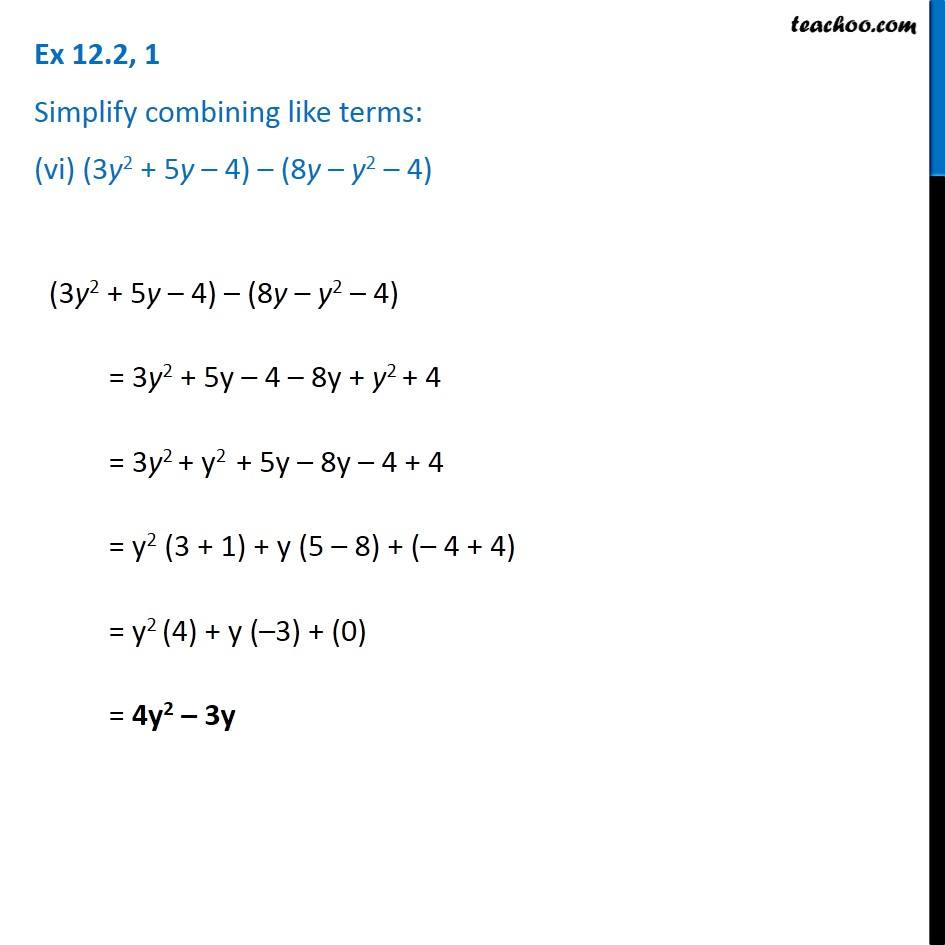Adding and Subtracting Like terms - Worksheet

Chapter 10 Class 7 Algebraic Expressions
Serial order wiseLearn in your speed, with individual attention - Teachoo Maths 1-on-1 Class

### Transcript

Question 1 Simplify combining like terms: (vi) (3y2 + 5y – 4) – (8y – y2 – 4) (3y2 + 5y – 4) – (8y – y2 – 4) = 3y2 + 5y – 4 – 8y + y2 + 4 = 3y2 + y2 + 5y – 8y – 4 + 4 = y2 (3 + 1) + y (5 – 8) + (– 4 + 4) = y2 (4) + y (–3) + (0) = 4y2 – 3y